# Subtracting + multiplication - math problems

#### Number of problems found: 50

• How manzBy how many is the product of the numbers 328 and 7 greater than its sum?
• Numbers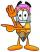By how many is the difference of numbers 8 and 34 less than its product?How much and how many times is 72.1 greater than 0.00721?
• Operations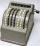Sum of the numbers 1.01 and 3.35 multiply by the difference of numbers 6.69 and 1.39.
• EmployeesOf all 360 employees, there are 11/12 women. How many men work in a company?
• Evaluate expressionIf x=2, y=-5 and z=3 what is the value of x-2y
• In the bowlThere are 12 pears in the bowl. Erik ate a third. How many pears remained in the bowl?
• Expression 6Evaluate expression: -6-2(4-8)-9
• Brackets 2Add parenthesis to make true: 5-2×6-4+2=5
• With bracket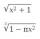Calculate (evaluate) simple mathematical expression with a negative numbers and a bracket: 13+15*5-2*(-6)
• Saving moneyMarko saved € 64. Milan saved 8 euros less than Marko. Marika saved 6 times more than Milan. Calculate who saved how much.
• Three-digit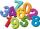How many three-digit natural numbers is greater than 321 if no digit in number repeated?
• Product of the sum and differenceCalculate the product of the sum and difference of numbers -7 and -2.
• RootThe root of the equation ? is (equal or greater or less than zero)? ?
• Locomotive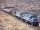Whole loaded train weighs 760 tons. It has twenty wagons, each carrying 20 tons of grain and empty weighs 10 tons. How much weight a locomotive?
• EricaErica bought 3 1/2 yards of fabric. If she uses 2/3 of the fabric, how much will she have left?
• Expression 1What is 7+8-(5×2)+5-4+(6×(5-3)+6)-(8+10)-7+6?
• Algebra problemThis is algebra. Let n represent an unknown number. 1. Eight more than the number n 2. Three times the number n 3. The product of the number n and eight 4. Three less than the number n 5. Three decreased by the number n
• Katy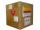Kate paid for the package on delivery of four meters textile 688 CZK. What is the price of one meter textile when shipping and handling amounted to 48 CZK?
• Cake fractions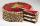Thomas ate 1/3 of cake, Bohouš of the rest of the cake ate 2/5. What fraction of cake left over for others?

Do you have an interesting mathematical word problem that you can't solve it? Submit a math problem, and we can try to solve it.

We will send a solution to your e-mail address. Solved examples are also published here. Please enter the e-mail correctly and check whether you don't have a full mailbox.

Please do not submit problems from current active competitions such as Mathematical Olympiad, correspondence seminars etc...